```SELECTED PRINCIPLES: ELECTROLYSIS (1)
Electrolytes are substances which contain free-moving ions; examples
include molten salts, and aqueous solutions of acids, bases, and salts.
And electrolysis is the decomposition of an electrolyte by an electric
current, as a result of redox reactions which occur at electrodes.
The synthesis of aqueous zinc bromide is an exergonic process; i.e.,```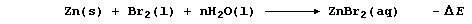```Not surprisingly, therefore, but with a delightful feeling of logic,
the decomposition of aqueous zinc bromide by an electric current is an
endergonic process; i.e.,```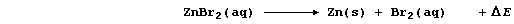```The diagram shows an external circuit connected to an electrolytic cell
consisting of two carbon-graphite electrodes immersed in an aqueous
solution of zinc bromide; the ions present are Zn2+(aq), Br1-(aq), and,
as a result of the ionization of water, H1+(aq) and OH1-(aq).```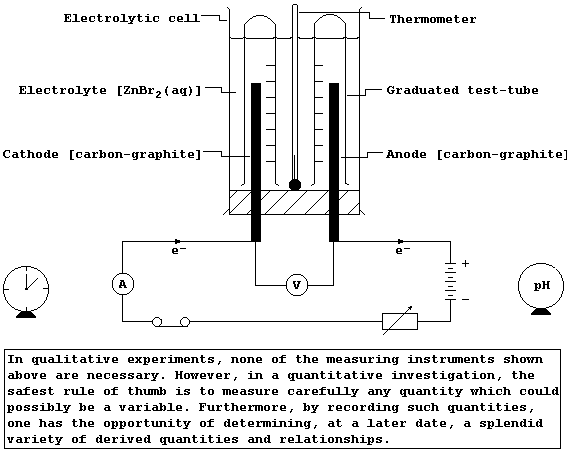```When a small, direct electric current is passed through this (initially
colourless) electrolyte, an orange-brown solution is formed around the
electrode connected to the positive side of the battery, and a grey
solid is deposited on the electrode connected to its negative side;
these observations are interpreted as follows.
The negatively charged bromide anions migrate to the positively charged
anode, where they are discharged by losing electrons; i.e.,```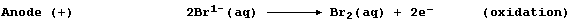```These electrons are forced round the external circuit to the cathode,
by the electrical energy transduced from the battery's chemical energy.
The positively charged zinc cations migrate to the negatively charged
cathode, where they are discharged by gaining electrons; i.e.,```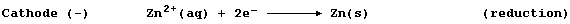```1.  Explain why solid ionic compounds are non-electrolytes. ___________
_______________________________________________________________________
_______________________________________________________________________

2.  The synthesis of (liquid) water can be summarized by this equation:```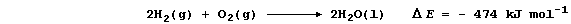```Construct a similar equation for the decomposition of (liquid) water.
_______________________________________________________________________

3.  In principle, the ease of discharge of ions from aqueous solutions
should correspond to their redox potentials: in practice, however,
other variables are known to be important. Because the details of these
complications are beyond the scope of this text, two predictive rules
of thumb are presented here (when 'inert' electrodes are used): first,
the anodic product is usually dioxygen, unless the electrolyte contains
halide ions; and second, the cathodic product is always a metal or
dihydrogen, and is usually this gas if the metal is more reactive than
zinc. The Table below summarizes the reactions of various electrolytes
at carbon graphite electrodes. Complete this Table, by inserting the
correct substance from this list: CuCl2(aq), HCl(aq), H2O(l), HNO3(aq),
H2SO4(aq), MgCl2(aq), MgCl2(l), MgCl2(s), MgSO4(aq), and ZnI2(aq).```
 `Substance` ` Reaction(s) at anode` ` Reaction(s) at cathode` ` ` ` None ` ` None ` ` ` ` 2Cl1-(aq) Ś« Cl2(g) + 2e-` ` Mg2+(aq) + 2e- Ś« Mg(l)` ` ` ` 2Cl1-(aq) Ś« Cl2(g/aq) + 2e-` ` 2H1+(aq) + 2e- Ś« H2(g)` ` ` ` 2I1-(aq) ŚŚ« I2(aq) + 2e-` ` Zn2+(aq) + 2e- Ś« Zn(s)` ` ` ` 2Cl1-(aq) Ś« Cl2(aq) + 2e-` ` Cu2+(aq) + 2e- Ś« Cu(s)` ` ` ` None ` ` None ` ` ` ` 2Cl1-(aq) Ś« Cl2(g/aq) + 2e-` ` 2H1+(aq) + 2e- Ś« H2(g)` ` ` ` 4OH1-(aq) Ś« O2(g) + 2H2O(l) + 4e-` ` 4H1+(aq) + 4e- Ś« 2H2(g)` ` ` ` 4OH1-(aq) Ś« O2(g) + 2H2O(l) + 4e-` ` 4H1+(aq) + 4e- Ś« 2H2(g)` ` ` ` 4OH1-(aq) Ś« O2(g) + 2H2O(l) + 4e-` ` 4H1+(aq) + 4e- Ś« 2H2(g)`
```                                                                    
Pure water conducts an electric current, albeit very weakly, because it
undergoes very slight ionization: nevertheless, there are too few ions
for significant electrolysis to occur. However, as the Table shows, the
electrolysis of water does occur when an ionic substance is present.
Suggest two more substances, one a soluble salt and the other an acid,
which would have a similar effect. ____________________________________

Dr. R. Peters                   Next                     Contents' List```